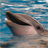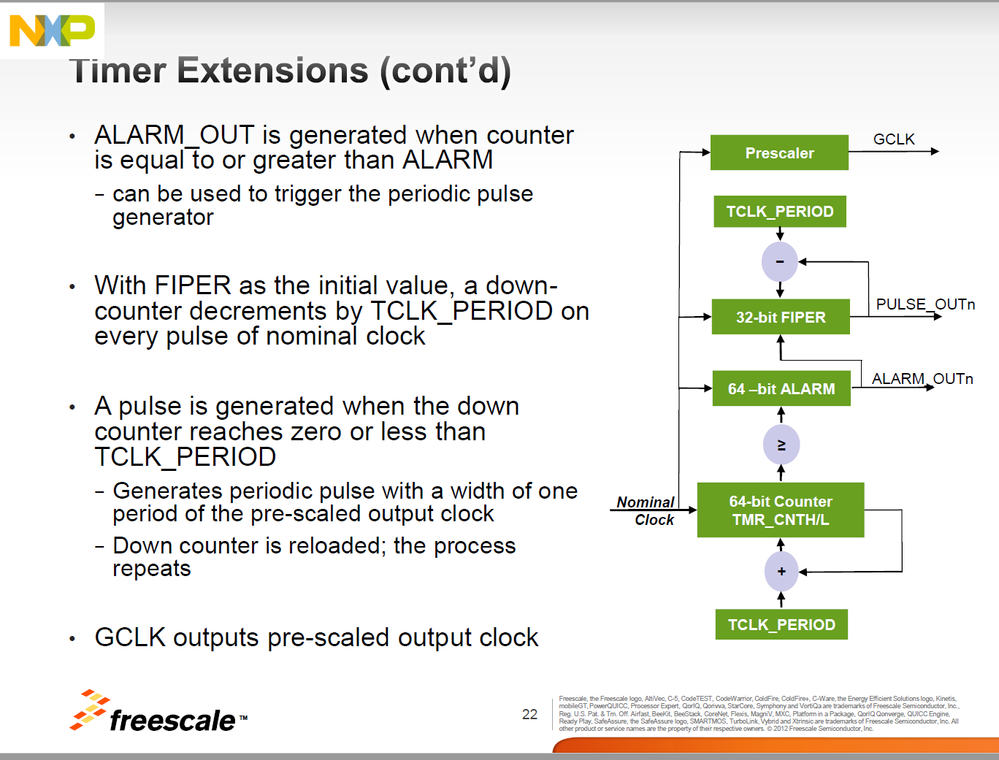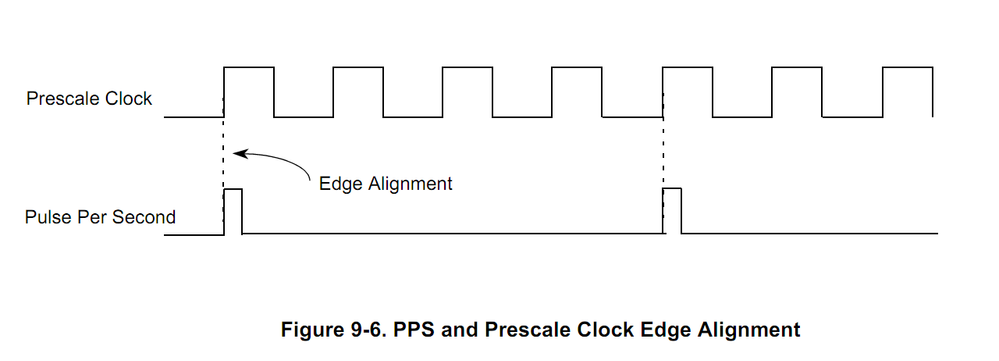1588: FIPER calculation

cancel
Showing results for
Did you mean:

1588: FIPER calculation

381 ViewsContributor I

I would like to calculate the FIPER counter initial value for 1588 purpose to get the proper pulse period at the output. Equation in DPAA Resource Manual  specification DPAA is

FIPER_VALUE = (prescale_value × tclk_per × N) – 1, or

TMR_FIPERn = (TMR_PRSC × TMR_CTRL[TCLK_PERIOD] × N)- 1

in the AN3423 the equation is :

Value of FIPER = (prescale_value × tclk_per × N) – tclk_per

Which is the good one?

ex: TCLK_PERIOD is 10 ns, the nominal frequency at the counter input is 100 MHz

I saw that in a NXP presentation that the pulse duration is equal to a period of GCLK (nominal clock / prescale). So increasing the prescale value allows to enlarge the pulse width...

Timing diagrams are missing in the NXP 1588 related documents.

So when the prescale value is 1 (GCLK = 100 MHz), If N = 6, I would get 10 ns pulse every 60 ns and FIPER = 59.

59 = (1 x 10 x 6) - 1

Can someone confirm?

If the prescale value = 2 , then GCLK = 50 MHz, my pulse will be 20 ns wide but the TCLK_PERIOD is still 10, so the FIPER counter decrements by 10 at nominal clock tick. How to set the FIPER value is I want a 20 ns pulse every 60ns?

It is said in the DPAA RM that:

It reloads the down counter in the cycle following a pulse.

So if N = 3, FIPER will be (2 x 10 x 3) - 1 = 59 but the counter will receive 6 pulse from 59 to 9 (decrements by TCLK_PPERIOD), the a 20 ns pulse will appears and same pattern will happen. But in this case the pulse period is no more 60 ns but 80 ns. If N= 2 then pulse period is 50ns.

Can someone confirm? my goal was 60ns!  Am I wrong or is there a missing information?

The example is with small number, my final goal is 1s but it is easier to draw timing with small number of period.

Is it sure that when the PRESC is different from 1, the steps of FIPER counter are only function of TCLK_PERIOD? Isn't PRESC involved in the FIPER step? What is the real equation involving the expected pulse period?

3 Replies
207 ViewsNXP TechSupport

Have a great day,

I suppose that you refer to the QorIQ Data Path Acceleration Architecture (DPAA) Reference Manual, Rev. 2.

At every tick of the timer accumulator overflow, the FIPER counter decrements by the value of TMR_CTRL[TCLK_PERIOD]. When the FIPER counter contains value less then TMR_CTRL[TCLK_PERIOD] it generates pulse on the next tick. Hence to keep tracking the prescaler output clock the FIPER_value can be in range

from (TMR_PRSC[PRSC_OCK] × TMR_CTRL[TCLK_PERIOD] × N) - 1

to     (TMR_PRSC[PRSC_OCK]  × TMR_CTRL[TCLK_PERIOD] × N) - TMR_CTRL[TCLK_PERIOD].

You did not point to NXP presentation you refer. For example FIPER pulse duration can be set identical to the width of the IEEE 1588 timer reference output clock on the devices with the QUICC Engine. IEEE 1588 Timer module described in the DPAA Reference Manual generates pulse width identical to the width selected timer clock. Please see Figure 5-15. Current Time Control in the DPAA manual. It clearly shows that FIPER counter is only function of TCLK_PERIOD and prescaler is not involved in the FIPER step.

-----------------------------------------------------------------------------------------------------------------------
-----------------------------------------------------------------------------------------------------------------------

207 ViewsContributor I

Have a great day too,

The presentation I refer to is FTF-NET-F0341 (2011) and information is the same in FTF-NET-F0102. It is about the FIPER pulse width. Please tell me if the information is correct or not. I attach an extract of the FTF-NET-F0102.You say that the  prescaler is not  involved in the FIPER counter but the prescaler is involved in the FIPER value as it part of the equation.

If the TMR_PRSC[PRSC_OCK] is 1, the equation is simplified:

• the pulse width is 1 period of "TMR_CNT clock",
• pulse period is N x TMR_CNT clock period.

If the TMR_PRSC[PRSC_OCK] greater than 1,

• the pulse width is GCLK period  of "TMR_CNT clock" (see presentation). or is it always the TMR_CNT clock period? Please tell the correct one.
• pulse period is  N x TMR_CNT clock period x ??? (how to choose N?)

After beginning of FIPER pulse, is the FIPER counter loaded with FIPER value at next tick of TMR_CNT clock ?

Must the term (TMR_PRSC[PRSC_OCK] × TMR_CTRL[TCLK_PERIOD] × N) represent the number of TMR_CNT clock period we want to get for the FIPER pulse period?

207 ViewsNXP TechSupport

I could find FTF-NET-F0102 only. It does not mention any nxp communication processor with DPAA. As I wrote there are nxp communications processors with the QE where Fiper pulse width can be determined according to the period of the selected timer input clock or according to the width of the timer reference output clock (GCLK). Some of them have TMR_CTRL[bit 4] to control that

0 PPS signal width is identical to the width selected timer clock (Default)

1 PPS signal width is identical to the width of the IEEE 1588 timer reference output clock

Other have this bit as reserved bit (for example MPC8360) and its PPS signal width is identical to the width selected input timer clock. Here corresponding figure from the QE manual.The DPAA manual says nothing for the pulse width. Also let assume the figure 15-5 I referred just does not show real fiper to prescaler bounds. In this case I recommend creating technical case and ask for the pulse width and how it is generated on particular communication processor. SeeHow I could create a Service Request?